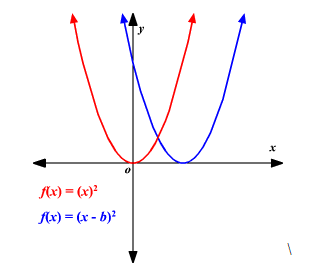# Transformations with Functions Worksheets

What is a Function Transformation? The transformation of functions is used to represent the functions in the form of a graph. It is as simple to understand as that. First, we need to understand what are the functions. A function represents the relation If you start with a simple parent function y=f(x)y=f(x) and its graph, certain modifications of the function will result in easily predictable changes to the graph. For example:Horizontal Shift - Replacing f(x) with f(x−b) results in the graph being shifted b units to the right between two or more values.Here are the important notes to keep in mind while using the function transformation. There is a vertical shift in the function if we add a constant to the output. There is a horizontal shift in the function if we add a constant to the input. We need to thoroughly examine the additions on the input or output side to compare and interpret vertical and horizontal shifts. Vertical and horizontal shifts often occur simultaneously.

• ### Basic Lesson

Guides students solving equations that involve transformations with functions. Demonstrates answer checking. Write the equation for the graph of function g(x), obtained by shifting the graph of f(x) = x2 four units left, stretching the graph vertically by a factor of three, reflecting that result over the xaxis, and then translating the graph down four units.

• ### Intermediate Lesson

Demonstrates how to solve more difficult problems. The graphs are exactly same. Functions f(-x) and f(x) are equal and graphs are symmetric with the y-axis. The functions are EVEN.

• ### Independent Practice 1

A really great activity for allowing students to understand the concept of transformations with functions. Write the equation for the graph of function g(x), obtained by shifting the graph of f(x) = x² two units left, stretching the graph vertically by a factor of four, reflecting that result over the x-axis, and then translating the graph up five units.

• ### Independent Practice 2

Students find the value of transformations with functions in assorted problems. The answers can be found below. Consider relationship between dollar and rupee. Graph these two functions on the same set of axes: y=x and y=(3/4)(x-4) Describe in transformational terms, how the first graph becomes the second graph.

• ### Independent Practice 3

A really great activity for allowing students to understand the concept of transformations with functions.

• ### Independent Practice 4

Students find the movement of transformations with functions in assorted problems. The answers can be found below.

• ### Homework Worksheet

Students are provided with problems to achieve the concepts of transformations with functions.

• ### Skill Quiz

This tests the students ability to evaluate Transformations with Functions.